# Appendix 2 - Calculational Procedure for 'Turn Time'

#### PhotoGrav 3.0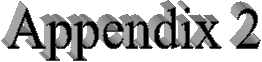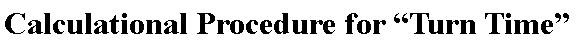A2.0 Introduction

This Appendix presents a procedure for calculating the “Turn Time” parameter which is used in the algorithm which estimates the time in seconds for an image to be engraved on your machine. See Section 3.6 for a brief description of the parameter and how it should be specified in the Machine Properties dialog window of the PhotoGrav program.

Section A2.1 describes a simple procedure which you can use to calculate the “Turn Time”. Section A2.2 describes a somewhat more complex procedure which you can use to calculate the “Turn Time” under more varied conditions so that you can perform some consistency checks on the calculational procedure. Section A2.3 then presents the model used to derive the calculational procedure and the rationale behind that model.

A2.1 Simple Procedure

This section describes a relatively simple procedure for calculating the “Turn Time” parameter.

Procedure

1. Prepare two grayshade images in a format that is acceptable for sending to your laser engraver. Make the first image a black square that is 1" wide (along scan) by 1" high (across scan). Make the other image a black rectangle that is 2" wide (along scan) by 1" high (across scan). Produce the images at the same resolution that you intend to use on the laser engraver, e.g., 250 dpi would probably be a good choice for a ULS machine and 300 dpi would probably be a good choice for an Epilog or LMI machine.A. To accomplish this in CorelDraw, start CorelDraw and create a new blank page as you would for any  engraving job. Select the rectangle tool and draw a rectangle exactly 1.00” tall x 1.00” wide, approximately centered on the page (turning the snap to grid on will make this easier). Select the pick tool and select the rectangle you just drew. Fill the rectangle with solid black by using the paint bucket tool. Set the outline to none by using the outline tool. Save this file as 1X1.CDR. Repeat the procedure and create a 1.00” tall x 2.00” wide image and save it as 1X2.CDR.B. To accomplish this in Adobe PhotoShop, start PhotoShop and set the Background color to black. Then, from the File menu, select New. In the dialog box that appears, set the Width to 1", the Height to 1", the Resolution to 250 or 300 pixels per inch, the Mode to Grayscale, and for the Contents select the option for "Background Color". Then click the "OK" command button. Save the image in any format that you can send to your engraver and give it a name so you can remember it as the 1" square. Repeat this process to create the 2" x 1" black image.

The images you have produced should appear as depicted in Fig. A2.1.1.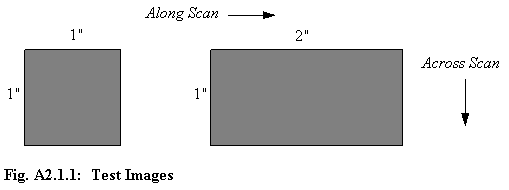2. Engrave each of the two images making sure that your engraver is in raster mode, not vector mode, and that the speed is set to its maximum value (100%). Also, set the engraver resolution, r, to the same value as that used to create both images, e.g., r = 250 dpi or r = 300 dpi. Record the time in seconds that it takes, as measured by your engraver, to complete the engraving. Designate the time to engrave the 1" x 1" square as T1 and the time to engrave the 2" x 1" rectangle as T2.

3. Calculate the "Turn Time" parameter, t, using the following formula:t = (2T1 - T2) / r where t = "Turn Time" parameter in seconds T1 = Time (in seconds) to engrave the 1" x 1" square T2 = Time (in seconds) to engrave the 2" x 1" rectangle r = Engraver & image resolution in dots per inch

For example, if T1= 46 seconds, T2 = 56 seconds, and r = 250 dots per inch, then t = 0.144 seconds and that is the number (0.144) which should be entered for the "Turn Time" parameter in the Machine Properties dialog window as specified in Section 3.6.

4. Perform the following check to see if the measured numbers make sense. Calculate, from the measured numbers, the maximum speed, s, for your engraver using the following formula:s = r / (T2 - T1) where s is measured in inches per second.

Using the same example numbers as those used in step 3, we get s = 25 inches per second for the maximum speed of the laser engraver used to generate the samples.

If, using the numbers you have measured, the value of s is not close to the maximum speed of your engraver as specified in your engraver documentation, then the value for the "Turn Time" parameter, t, as calculated in Step 3 is probably not reliable and any engraving time estimates which PhotoGrav produces are also not reliable. If engraving time estimates are important to you, then request assistance as specified in Chapter 4.

A2.2 More Complex Procedure

The procedure described in this section is actually not any more complex than the procedure described in Sec. A2.1 but it does require a few more variables for its specification. However, as a result, it is considerably more general than the previous procedure and will allow you to calculate the "Turn Time" parameter with higher precision and to perform consistency checks by measuring the parameter under several conditions.

Procedure

1. Prepare two grayshade images in a format that is acceptable for sending to your laser engraver. Make the first image a black rectangle that is w1 inches wide (along scan) by h1 inches high (across scan). Make the other image a black rectangle that is w2 inches wide (along scan) by h2 inches high (across scan). Produce the images at the same resolution that you intend to use on the laser engraver, e.g., 250 dpi would probably be a good choice for a ULS machine and 300 dpi would probably be a good choice for an Epilog or LMI machine. The images can be produced in CorelDraw or PhotoShop by small modifications to the procedures described in Sec. A3.1, Steps 1A and 1B. The images you have produced should appear as depicted in Fig. A2.2.1.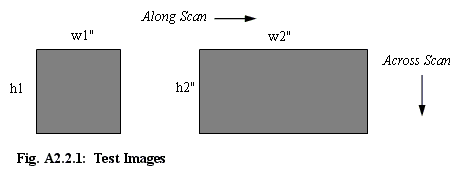2. Engrave each of the two images making sure that your engraver is in raster mode, not vector mode, and that the speed is set to its maximum value (100%). Also, set the engraver resolution, r, to the same value as that used to create both images, e.g., r = 250 dpi or r = 300 dpi. Record the time in seconds that it takes, as measured by your engraver, to complete the engraving. Designate the time to engrave the the first rectangle as T1 and the time to engrave the second rectangle as T2.

3. Calculate the "Turn Time" parameter, t, using the following formula:t = (h1 x w1 x T2 - w2 x h2 x T1) / ( (w1 - w2) x r x h1 x h2 ) where t = "Turn Time" parameter in seconds T1 = Time (in seconds) to engrave the first rectangle T2 = Time (in seconds) to engrave the second rectangle r = Engraver & image resolution in dots per inch

For example, if w1 = 1", h1 = 2", w2 = 4", h2 = 1", T1= 90 seconds, T2 = 75 seconds, and r = 250 dots per inch, then t = 0.140 seconds and that is the number (0.140) which should be entered for the "Turn Time" parameter in the Machine Properties dialog window as specified in Section 2.6.

4. Perform the following check to see if the measured numbers make sense. Calculate, from the measured numbers, the maximum speed, s, for your engraver using the following formula:s = ( r x (w2 - w1) x h1 x h2 ) / ( h1 x T2 - h2 x T1 ) where s is measured in inches per second.

Using the same example numbers as those used in step 3, we again get s = 25 inches per second for the maximum speed of the laser engraver used to generate the samples.

If, using the numbers you have measured, the value of s is not close to the maximum speed of your engraver as specified in your engraver documentation, then the value for the "Turn Time" parameter, t,

The procedure described in this section provides much more flexibility than the simple procedure described in Sec A2.1. For example, one can use larger rectangles and/or a larger value for the engraver and image resolutions to increase the precision of the calculated "Turn Time" parameter. Or one can use several pairs of different-sized rectangles, calculating the parameter independently from each pair, to determine the consistency of the calculated values. If you get inconsistent numbers for the parameter, or if the maximum speed, s, calculated above is not close to the manufacturer's published value, then request assistance as specified in Chapter 4.

A2.3 Calculational Model

Consider the rectangular black object in Fig. A2.3.1 which is an image which is to be engraved.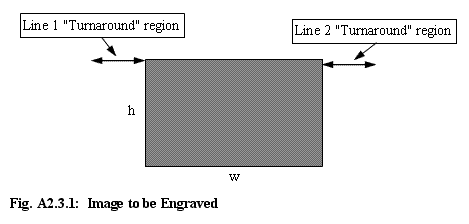The width of the rectangle is "w" inches and the height of the rectangle is "h" inches. The "along scan" direction is horizontal in Fig. A2.3.1 and the cross scan direction is vertical. Assume that the image was created with a resolution of "r" dots per inch (dpi) and that it will be engraved at that same resolution. The region designated "Line 1 Turnaround region" in Fig A2.3.1 is the distance that the engraver requires, after scanning Line 1, to decelerate, move down (1/r) inches, and to then accelerate to begin the scan for Line 2. There is one such region associated with every scan line to be engraved.

Assume that the time, "t", that the engraver spends in this region is independent of the engraving speed, "s", and is the same for every scan line. Then the time spent on each scan line is (w/s + t) seconds where s is specified in inches per second, w is specified in inches, and t is given in seconds. The number of scan lines in the rectangle of Fig. A2.3.1 is (r x h) where r is given in dots per inch and h is measured in inches. Thus, the total time, T, to engrave the rectangle is:T = (r x h) x (w/s + t)

Now assume that we have two rectangles to be engraved, one of dimensions w1 x h1 and the other of dimensions w2 x h2. The total times, T1 and T2, to engrave these rectangles are given by:T1 = (r x h1) x (w1/s + t) and T2 = (r x h2) x (w2/s + t)

These two equations can be regarded as two equations in two unknowns, s and t (although s is "known" from the manufacturer's literature, it provides a convenient check to regard it as an unknown). The two equations can be solved for t and s with the following solutions:t = (h1 x w1 x T2 - w2 x h2 x T1) / ( (w1 - w2) x r x h1 x h2 ) s = ( r x (w2 - w1) x h1 x h2 ) / (h1 x T2 - h2 x T1)

which are exactly the formulas used in Sec. A2.2. Note that the speed, s, in these equations need not be the maximum speed of the engraver. That assumption was made in the preceding sections to simplify the procedures and explanations.

Obviously, the "Simple Procedure" described in Section A2.1 could be simplified even more by assuming that one does indeed know the speed of the engraver and then substituting that value of s directly in one of the two equations and solving for t directly. In that case, one need only make and engrave one rectangular image to determine t but this ultra simple procedure provides no consistency check at all.

The algorithm within PhotoGrav that calculates estimated engraving times is reasonably sophisticated in that it knows and uses the actual length of each line to be engraved (first "burn" to last "burn") and will not "time" regions for which no engraving is to be done. However, there is a risk in that sophistication in that, if the "printer" drivers used by a manufacturer are less sophisticated in controlling the engraver, then PhotoGrav's time estimates could differ substantially from actual engraving times for that particular engraver.